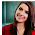# Computer System Architecture Set 16

### Questions 151 to 160

151.
Which of the following binary number is equivalent to 20?
 (a) 10100 (b) 100 (c) 10 (d) 11111 (e) 10111
152.
 (a) 10 (b) 2 (c) 8 (d) 16 (e) 5
153.
What is equivalent of -3510 in 8-bit 2’s complement representation?
 (a) 1.0111e+06 (b) 1.10111e+07 (c) 1.10111e+07 (d) 101101 (e) 1.10011e+07
154.
The BCD equivalent of decimal number 32.94 is
 (a) 0011 0010 . 1001 0100 (b) 1011 0010 . 1001 0100 (c) 0011 0010 . 1001 0010 (d) 100010 . 1001 0100 (e) 0010 0010 . 1001 0010.
155.
The code which can represent numbers, characters, and special characters are called
 (a) Gray code (b) BCD code (c) EBCDIC code (d) Alphanumeric code (e) ASCII code.
156.
In a Hamming code for transmitting a data of 4-bit, how many parity bit(s) is/are used?
 (a) One (b) Two (c) Three (d) Four (e) Zero.
157.
The Boolean expression F = AB’C’D + AB’CD’ + A’BCD can be written as
 (a) F = ∑m (7, 8, 9) (b) F = ∑m (7, 9, 10) (c) F = ∑m (7, 8, 10) (d) F = ∑m (7, 6, 10) (e) F = ∑m (6, 9, 10).
158.
The Boolean expression F(A,B,C,D) =m(0, 3) is
 (a) (A + B + C’ + D’) (A + B + C + D) (b) (A + B’+ C’ + D’) (A’+ B’+ C’ + D’) (c) (A’+ B’+ C’ + D’) (A’+ B’+ C  + D) (d) (A + B’ + D’) (A’ + B + C + D’) (e) (A + B + D’) (A’ + B’ + C + D’).
159.
In a Karnaugh map the adjacent minterms can be combined only if the configuration is
 (a) 2 (b) 4 (c) 2 x n (d) 2n (e) 2n-1.
160.
For realizing the Boolean expression AC + AD + BC + BD how many  number of inputs are needed ?
 (a) 8 (b) 4 (c) 12 (d) 16 (e) 2

1.